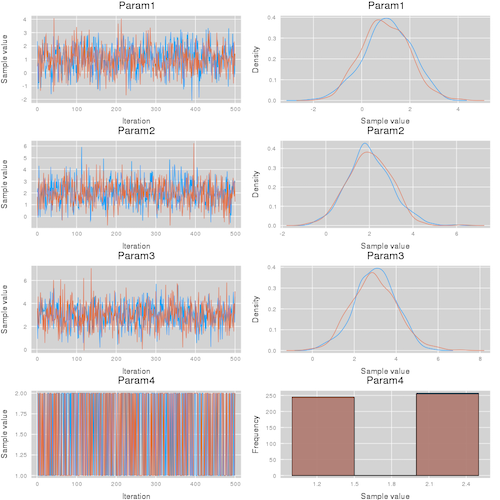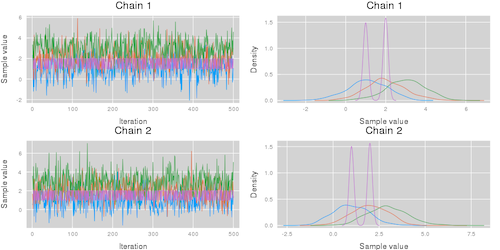Skip to content

# TuringLang/MCMCChains.jl

Chain types and utility functions for MCMC simulations
Julia
Latest commit 94c696a Aug 9, 2019
Type Name Latest commit message Commit time
Failed to load latest commit information.src Aug 5, 2019test Aug 3, 2019.gitignore Aug 20, 2018.travis.yml Sep 20, 2018LICENSE.md Aug 20, 2018Project.toml Aug 9, 2019README.md Jul 12, 2019REQUIRE Mar 26, 2019appveyor.yml Aug 20, 2018

# MCMCChains.jl

Implementation of Julia types for summarizing MCMC simulations and utility functions for diagnostics and visualizations.

## Example

The following simple example illustrates how to use Chain to visually summarize a MCMC simulation:

```using MCMCChains
using StatsPlots

theme(:ggplot2);

# Define the experiment
n_iter = 500;
n_name = 3;
n_chain = 2;

# experiment results
val = randn(n_iter, n_name, n_chain) .+ [1, 2, 3]';
val = hcat(val, rand(1:2, n_iter, 1, n_chain));

# construct a Chains object
chn = Chains(val);

# visualize the MCMC simulation results
p1 = plot(chn)
p2 = plot(chn, colordim = :parameter)
```

This code results in the visualizations shown below. Note that the plot function takes the additional arguments described in the Plots.jl package.

Summarize parameters  Summarize chains
`plot(chn; colordim = :chain)` `plot(chn; colordim = :parameter)`## Manual

### Chains type

```# construction of a Chains object with no names
Chains(val::AbstractArray{A,3};
start::Int=1,
thin::Int=1,
evidence = 0.0,
info=NamedTuple())

# construction of a chains object with new names
Chains(val::AbstractArray{A,3},
parameter_names::Vector{String},
name_map = copy(DEFAULT_MAP);
start::Int=1,
thin::Int=1,
evidence = 0.0,
info=NamedTuple())

# Indexing a Chains object
chn = Chains(...)
chn_param1 = chn[:,2,:] # returns a new Chains object for parameter 2
chn[:,2,:] = ... # set values for parameter 2```

### Parameter Names

Chains can be constructed with parameter names, like so:

```# 500 samples, 5 parameters, two chains.
val = rand(500,5, 2)

chn = Chains(val, ["a", "b", "c", "d", "e"])```

By default, parameters will be given the name `Parami`, where `i` is the parameter number.

### Sections

Chains parameters are sorted into sections, which are types of parameters. By default, every chain contains a section called `:parameters`, which is where all values are assigned unless assigned elsewhere. Chains can be assigned a named map during construction:

```chn = Chains(val,
["a", "b", "c", "d", "e"],
Dict(:internals => ["d", "e"]))```

Or through the `set_section` function, which returns a new `Chains` object (as `Chains` objects cannot be modified in place due to section map immutability):

`chn2 = set_section(chn, Dict(:internals => ["d", "e"]))`

Any parameters not assigned will be placed into `:parameters`.

Calling `show(chn)` provides the following output:

```Log evidence      = 0.0
Iterations        = 1:500
Thinning interval = 1
Chains            = 1, 2, 3
Samples per chain = 500
parameters        = c, b, a

Empirical Posterior Estimates
────────────────────────────────────
parameters
Mean    SD   Naive SE  MCSE  ESS
a 0.5169 0.2920   0.0075 0.0066 500
b 0.4891 0.2929   0.0076 0.0070 500
c 0.5102 0.2840   0.0073 0.0068 500

Quantiles
────────────────────────────────────
parameters
2.5%   25.0%  50.0%  75.0%  97.5%
a 0.0001 0.2620 0.5314 0.7774 0.9978
b 0.0001 0.2290 0.4972 0.7365 0.9998
c 0.0004 0.2739 0.5137 0.7498 0.9997```

Note that only `a`, `b`, and `c` are being shown. You can explicity show the `:internals` section by calling `describe(chn, sections=:internals)` or all variables with `describe(chn, showall=true)`. Most MCMCChains functions like `plot` or `gelmandiag` support the `section` and `showall` keyword arguments.

### The `get` Function

MCMCChains provides a `get` function designed to make it easier to access parameters `get(chn, :P)` returns a `NamedTuple` which can be easy to work with.

Example:

```val = rand(500, 5, 1)
chn = Chains(val, ["P", "P", "P", "D", "E"]);

x = get(chn, :P)```

Here's what `x` looks like:

`(P = (Union{Missing, Float64}[0.349592; 0.671365; … ; 0.319421; 0.298899], Union{Missing, Float64}[0.757884; 0.720212; … ; 0.471339; 0.5381], Union{Missing, Float64}[0.240626; 0.987814; … ; 0.980652; 0.149805]),)`

You can access each of the `P[. . .]` variables by indexing, using `x.P`, `x.P`, or `x.P`.

`get` also accepts vectors of things to retrieve, so you can call `x = get(chn, [:P, :D])`. This looks like

```(P = (Union{Missing, Float64}[0.349592; 0.671365; … ; 0.319421; 0.298899], Union{Missing, Float64}[0.757884; 0.720212; … ; 0.471339; 0.5381], Union{Missing, Float64}[0.240626; 0.987814; … ; 0.980652; 0.149805]),
D = Union{Missing, Float64}[0.648963; 0.0419232; … ; 0.54666; 0.746028])```

Note that `x.P` is a tuple which has to be indexed by the relevant index, while `x.D` is just a vector.

### Convergence Diagnostics functions

#### Discrete Diagnostic

Options for method are `[:weiss, :hangartner, :DARBOOT, MCBOOT, :billinsgley, :billingsleyBOOT]`

`discretediag(c::AbstractChains; frac=0.3, method=:weiss, nsim=1000)`

#### Gelman, Rubin, and Brooks Diagnostics

`gelmandiag(c::AbstractChains; alpha=0.05, mpsrf=false, transform=false)`

#### Geweke Diagnostic

`gewekediag(c::AbstractChains; first=0.1, last=0.5, etype=:imse)`

#### Heidelberger and Welch Diagnostics

`heideldiag(c::AbstractChains; alpha=0.05, eps=0.1, etype=:imse)`

#### Raftery and Lewis Diagnostic

`rafterydiag(c::AbstractChains; q=0.025, r=0.005, s=0.95, eps=0.001)`

### Plotting

```# construct a plot
plot(c::AbstractChains, seriestype = (:traceplot, :mixeddensity))

# construct trace plots
plot(c::AbstractChains, seriestype = :traceplot)
# or for all seriestypes use the alternative shorthand syntax
traceplot(c::AbstractChains)

# construct running average plots
meanplot(c::AbstractChains)

# construct density plots
density(c::AbstractChains)

# construct histogram plots
histogram(c::AbstractChains)

# construct mixed density plots
mixeddensity(c::AbstractChains)

# construct autocorrelation plots
autocorplot(c::AbstractChains)

# make a cornerplot (requires StatPlots) of parameters in a Chain:
corner(c::AbstractChains, [:A, :B])```

### Saving and Loading Chains

Chains objects can be serialized and deserialized using `read` and `write`.

```# Save a chain.
write("chain-file.jls", chn)

# Read a chain.
chn2 = read("chain-file.jls", Chains)```

### Exporting Chains

A few utility export functions have been provided to convers `Chains` objects to either an Array or a DataFrame:

```# Several examples of creating an Array object:
Array(chns)
Array(chns[:s])
Array(chns, [:parameters])
Array(chns, [:parameters, :internals])

# By default chains are appended. This can be disabled
# using the append_chains keyword argument:
Array(chns, append_chains=false)

# This will return an `Array{Array, 1}` object containing
# an Array for each chain.

# A final option is:
Array(chns, remove_missing_union=false)

# This will not convert the Array columns from a
`Union{Missing, Real}` to a `Vector{Real}`.```

Similarly, for DataFrames:

```DataFrame(chns)
DataFrame(chns[:s])
DataFrame(chns, [:parameters])
DataFrame(chns, [:parameters, :internals])
DataFrame(chns, append_chains=false)
DataFrame(chns, remove_missing_union=false)```

See also ?MCMCChains.DataFrame and ?MCMCChains.Array for more help.

### Sampling Chains

MCMCChains overloads several `sample()` methods as defined in StatsBase:

```# Sampling `n` samples from the chain `a`. Optionally
# weighting the samples using `wv`.
sample([rng], a, [wv::AbstractWeights], n::Integer)

# E.g. creating 10000 weighted samples:
c = kde(Array(chn[:s]))
chn_weighted_sample = sample(c.x, Weights(c.density), 100000)

# As above, but supports replacing and ordering.
sample([rng], a, [wv::AbstractWeights], n::Integer; replace=true,
ordered=false)```

See also ?MCMCChains.sample for additional help.

## License Notice

Note that this package heavily uses and adapts code from the Mamba.jl package licensed under MIT License, see License.md.

You can’t perform that action at this time.